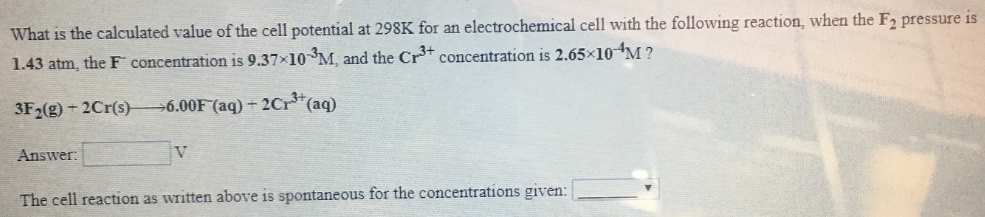# What is the calculated value of the cell potential at 298K for an electrochemical cell with the following reaction, when the F2 pressure is 1.43 atm, the F^- concentrations is 9.37x10^-3M, and the Cr^3+ concentrations is 2.65x10^-4M? 3F2(g) + 2Cr(s) → 6.00F^-(aq) + Cr^3+(aq) Answer: ____ V The cell reaction as written above is spontaneous for the concentrations given: ____